# Two-step equations

##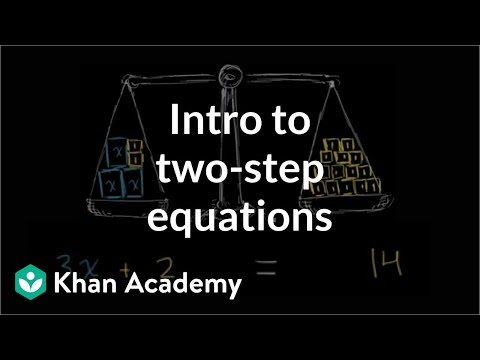By Khan Academy

Here's how we solve a two step equation. It begins with the concept of equality: what we do to one side of the equation must be done to the other.# Two-step equations

##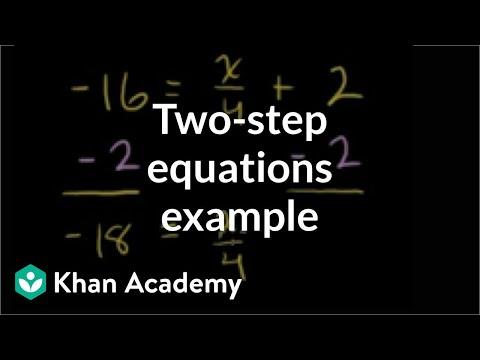By Khan Academy

Solve the equation by isolating the term with numerator x.# Two-step equations

##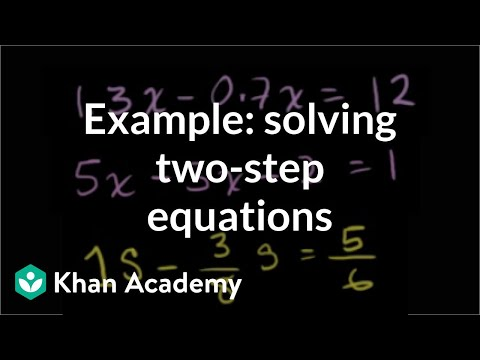By Khan Academy

Let's practice some two step equations, some of which require merging terms and using the distributive property.# Two-step equations

##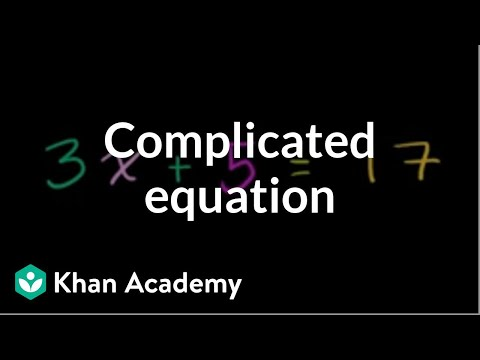By Khan Academy

This example demonstrates how we solve an equation expressed such: ax + b = c. It's a little more complicated than previous examples, but you can do it!# Linear equation word problems

##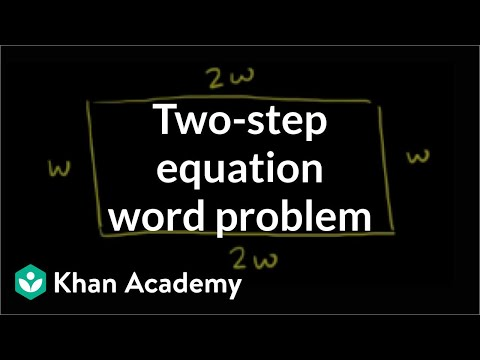By Khan Academy

Here's a nifty word problem in which we find the dimensions of a garden given only the perimeter. Let's create an equation to solve!# Linear equation word problems

##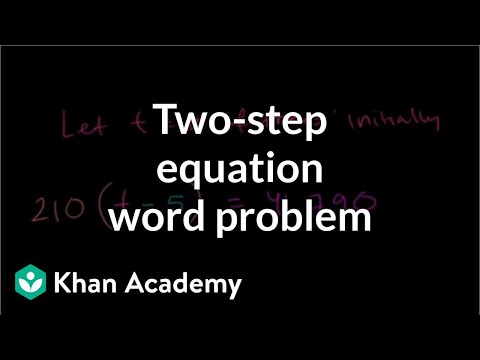By Khan Academy

Learn how to solve a word problem by writing an equation to model the situation. In this video, we use the linear equation 210(t-5) = 41,790.# Two-step equations with decimals and fractions

##By Khan Academy

Learn how to solve equations that involve decimals and fractions. The equations shown in this video are called two-step equations because they each take two steps to solve.# 7.ee.4a

##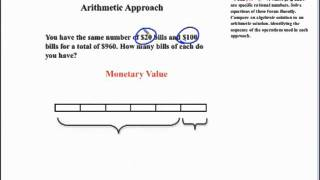By SEDL

This mathematics video is from the Common Core State Standards Video Series at http://secc.sedl.org/common_core_videos/# Word Problem Involving the Perimeter of a Rectangle – Ex 1

##By PatrickJMT

YouTube presents Word Problem Involving the Perimeter of a Rectangle, an educational video resource on math.# Word Problem Involving Perimeter of a Triangle - ex 2

##By PatrickJMT

YouTube presents Word Problem Involving Perimeter of a Triangle - ex 2, an educational video resource on math.# Constructing and solving a multi-step inequality example

##By Khan Academy

This video lecture series on Worked Examples in Algebra from Khan Academy includes Solving Equations Solving Word Problems Solving for a variable Absolute Value and Number Lines Patterns in Sequences Functional Relationships Domain and Range Rate Problems Linear Functions Slope of a Line X and Y intercepts Equation of a Line Parallel Lines Perpendicular Lines Solving Inequalities and more...# Finding Slope Given Graph and Two Points (Part 2)

##By Khan Academy

This video lecture series on Algebra from Khan Academy includes Linear Equations Solving Inequalities Slope and Y-intercept Equation of a Line Averages Integer sums Taking Percentages Systems of Equations Ratios Multiplying Expressions Solving a quadratic by factoring Imaginary Numbers Complex Numbers Quadratic Equations Quadratic Inequalities Functions Conic sections Circles Ellipses Hyperbolas......# Grade 7 Math - Modeling Using Equations or Inequalities

##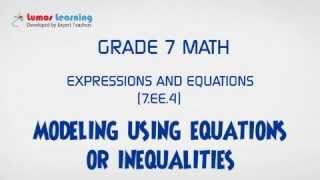By Lumos Learning

Using the Lumos Study Programs, parents and educators can reinforce the classroom learning experience for children and help them succeed at school and on the standardized tests. Lumos books, dvd, eLearning and tutoring are used by leading schools, libraries and thousands of parents to supplement classroom learning and improve student achievement in the standardized tests.